## An Introduction to Graphing in Python¶

The core plotting library in Python is matplotlib. The matplotlib gallery is a great way to figure out how to make the kind of plots you want. Just look for a plot of the right basic style and click on the image to see the code that generated it.

Two things that may differ a bit from other graphing programs that you are used to:

• Making a plot will return a plot object. If you assign this to something you can use it to modify the figure later. This is why in some of the examples below you will see some output before the figure.
• In some Python interpreters you will need to explicitly tell Python to display the figures. This is done using the show() function. Several examples of using show() are included below.

## Basic plots of two variables¶

Generating basic bivariate plots is done using the plot() function.

In :
import matplotlib.pyplot as plt
import numpy as np

#generate some data
x = np.array(range(20))
y = 3 + 0.5 * x + np.random.randn(20)

#plot the data
plt.plot(x, y, 'bo')
plt.show()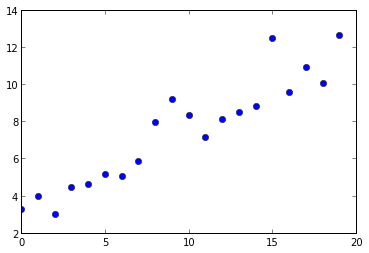We can also scale any of the axes logarithmically.

In :
fig = plt.loglog(x, y, 'rs')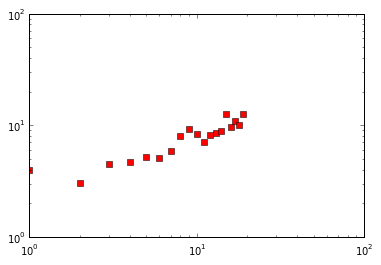In :
fig = plt.semilogx(x, y, 'g^')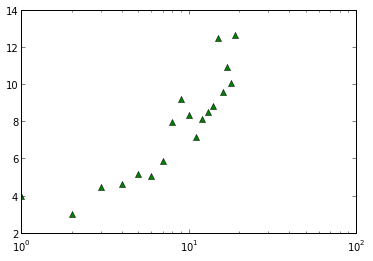## Histograms¶

Histograms are made using the hist() function.

In :
#generate some random numbers from a normal distribution
data = 100 + np.random.randn(500)

#make a histogram with 20 bins
plt.hist(data, 20)
plt.show()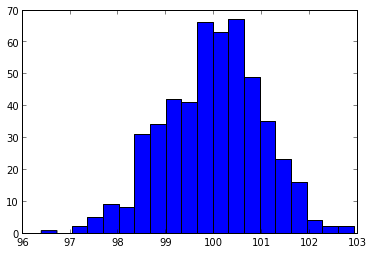## Labels¶

We can add axis labels to a figure using the xlabel() and ylabel() functions.

In :
plt.hist(data, 20)
plt.xlabel('Body Mass (g)', fontsize=20)
plt.ylabel('Number of Individuals', fontsize= 20)

Out:
<matplotlib.text.Text at 0x2bba450>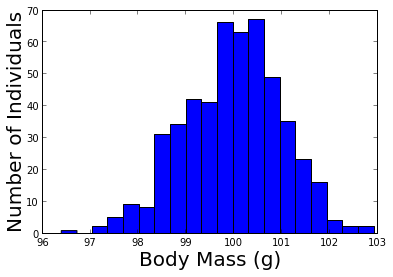## Axis Limits¶

Axis limits are changed using the axis([xmin, xmax, ymin, ymax]) function.

In :
plt.hist(data, 20)
plt.axis([90, 110, 0, 100])

Out:
[90, 110, 0, 100]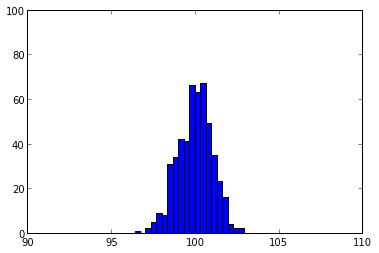## Plotting multiple sets of data together¶

To plot multiple datasets together we tell Python not to overwrite the previous data using hold(True). Running hold(False) will cause Python to start overwriting the figure again.

In :
x = np.array(range(20))
y = 3 + 0.5 * x + np.random.randn(20)
z = 2 + 0.9 * x + np.random.randn(20)

#plot the data
plt.plot(x, y, 'bo')
plt.hold(True)
plt.plot(x, z, 'r^')
plt.show()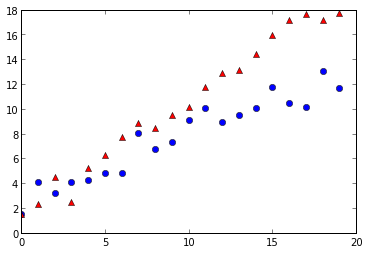## Subplots¶

Subplots are generated using subplot(#ofRows, #ofCols, Position).

In :
plt.subplot(1, 2, 1)
plt.plot(x, y, 'rs')
plt.subplot(1, 2, 2)
plt.hist(data, 10)
plt.show()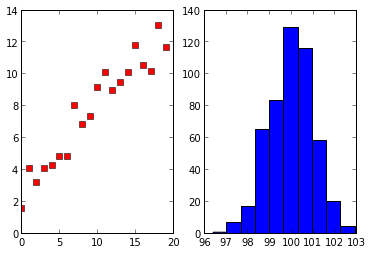## New Figures¶

Plotting multiple figures in the same script requires that we create new figures, which is done using figure(). In this example the two figures are different figures rather than subplots of a single figure.

In :
plt.plot(z, x, 'go')
plt.figure()
plt.plot(z, y, 'rs')

Out:
[<matplotlib.lines.Line2D at 0x3eb4710>]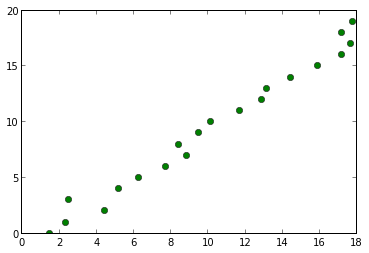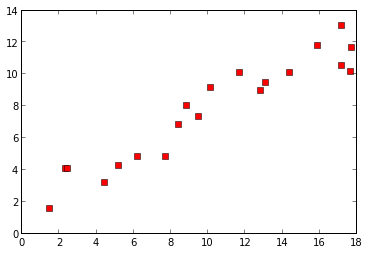## Want to know more?¶

Matplotlib is very powerful and there are lots of functions and arugments to create different kinds of figures and modify them as much as you would like. Check out the website or the gallery to learn more.